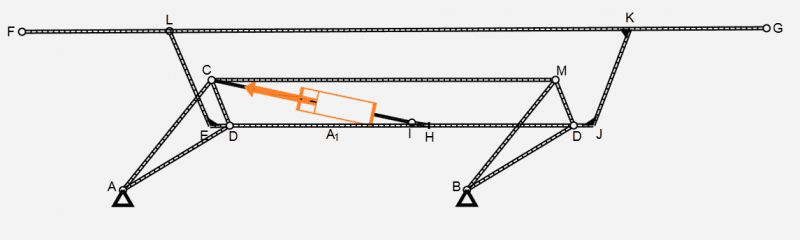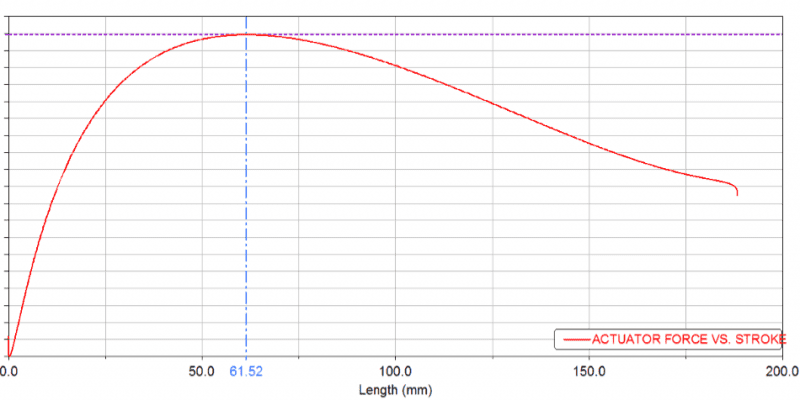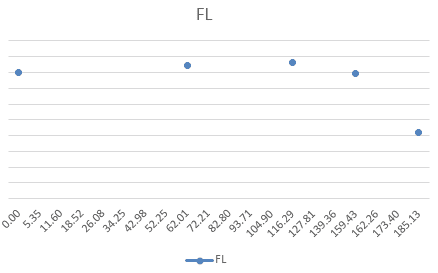# Static load Vs Push force on an actuator

Hello all,

I have an actuator to lift a table, with certain amount of wait on it.
Since I have mounted the actuator in an angle, I had to analyze the load on the actuator.
However, the velocity at which I am lifting the table is bare minimum compared to the load on the table. So I had the feeling that the static analysis would be sufficient to find the load on the actuator, therefore choose the appropriate actuator.

After getting the static load, through finite element analysis just for my confidence I ran the simulation for the complete articulation of the table using kinematic analysis. In theory I was expecting the values to be almost same. But the kinematic analysis gave more than 1.5 times the load that was observed in static analysis.

Shouldn't the force values be the same? Since acceleration is very less, the push force required by the actuator be just above the static load that is acting on it? Where am I going wrong in physics?

CWatters
Homework Helper
Gold Member
Since I have mounted the actuator in an angle, I had to analyze the load on the actuator.
However, the velocity at which I am lifting the table is bare minimum compared to the load on the table. So I had the feeling that the static analysis would be sufficient to find the load on the actuator, therefore choose the appropriate actuator.

That is a reasonable assumption.

After getting the static load, through finite element analysis just for my confidence I ran the simulation for the complete articulation of the table using kinematic analysis. In theory I was expecting the values to be almost same. But the kinematic analysis gave more than 1.5 times the load that was observed in static analysis.

Shouldn't the force values be the same? Since acceleration is very less, the push force required by the actuator be just above the static load that is acting on it? Where am I going wrong in physics?

We cannot say where you have made a mistake unless you provide a drawing and your calculations.

Hello CWatters,

Since the system has too many unknowns for the free body diagram to be resolved, we have used tools like adams(for kinematic analysis) and abaqus(for static analysis) to solve the problem.

I have pasted the basic line diagram below, on how the system looks like. But speaking theoretically, assuming no losses due to friction. The work done by the actuator to lift the table, shouldn't it be only to lift the static load?In the diagram, points: M,C,D,A ,B and I are pin joints. Rest are welded(means that the relative angle is fixed) Load acts on the link FG.

Would it be because the actuator is only partially under the load path?

Hello CWatters,

Since the system has too many unknowns for the free body diagram to be resolved, we have used tools like adams(for kinematic analysis) and abaqus(for static analysis) to solve the problem.

I have pasted the basic line diagram below, on how the system looks like. But speaking theoretically, assuming no losses due to friction. The work done by the actuator to lift the table, shouldn't it be only to lift the static load?
View attachment 78840

In the diagram, points: M,C,D,A ,B and I are pin joints. Rest are welded(means that the relative angle is fixed) Load acts on the link FG.
There is a load and an inertial component in the kinematic simulation vs the static. So if you could try to set the initial acceleration 10x lower in the kinematic to see if the result is a 1.05 of the static.

The structure is very position dependent, if your starting position is not the same (to the 0.1mm) you can get very different results in your starting setup, as your actuating force varies along the whole actuator travel length.

Other than that, you could check the link masses between the two.

There is a load and an inertial component in the kinematic simulation vs the static. So if you could try to set the initial acceleration 10x lower in the kinematic to see if the result is a 1.05 of the static.

The acceleration is far lower than 10x, and the inertia of the components is less than 20% of the load acting on the table. So, I was expecting a difference between the kinematic(which takes inertia n acceleration into account) but not as high as greater than 1.5 times.

I am certain about the position I have taken to start by kinematic analysis. Moreover, I have taken 5 different positions for static analysis. The force against the position is different for both the analysis report.The acceleration is far lower than 10x, and the inertia of the components is less than 20% of the load acting on the table. So, I was expecting a difference between the kinematic (which takes inertia n acceleration into account) but not as high as greater than 1.5 times.
I meant try to set the acceleration 10 times lower than the value used for the above graph to see what happens, you can compare the graphs afterwards.

The graphs could use some values (I don't know what to compare with what) and I would like to know is in what position the table is when the force of the kinematic peaks so at 62 piston travel. Is it the DCH 90 deg angle situation?
Also is the end of the graph the singular position where points CDH are collinear? (you have double D in your drawing)

Btw. For nomenclatural ease we keep calling it kinematic with forces, but these two are not very compatible. Kinetics should be better, or kinematics with accelerations, just the load on the piston is nonsensical in the latter case. Anyway be sure you are not comparing accelerations in one graph with forces in the other.

The graph what you see here is plotted for a constant velocity of 4.4mm/s
The actuator is only capable of providing a constant velocity. The only acceleration component is the gravity itself.

Link CD becomes perpendicular to the horizontal plane, static analysis shows maximum force at this position, however kinetics show peak value at a different position.

(My bad in naming the nodes incorrectly. )

I will take your point seriously and run the FEA again, considering the acceleration due to gravity. Or run the kinematic analysis without acceleration.

Link CD becomes perpendicular to the horizontal plane, static analysis shows maximum force at this position, however kinetics show peak value at a different position.
Well the static sounds just too good to be true with it's perpendicular to horizontal situation, considering you have 2 torques of almost arbitrary angles that will just not add up that nice.

As always it's the initial setup. But finding what is wrong is hard by just looking at the results.
Try and modify masses, inertial momentae and see what the results are doing, as it might be that one of your analyses is ignoring something (I'm betting on the static).

•MayZ91
I ran the kinematic analysis again, this time without using the gravity and the effect of load on the table is converted to the force(which is the exact scenario as taken for FEA). And I found the actuator vs force. The curve pattern remains the same, only the magnitude drops.

As always it's the initial setup. But finding what is wrong is hard by just looking at the results.
Try and modify masses, inertial momentae and see what the results are doing, as it might be that one of your analyses is ignoring something (I'm betting on the static).

From the above observation, with same boundary conditions(no inertial effect), I am still not able to find the match(not even the load curve let alone the magnitude).